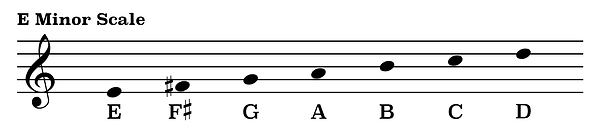top of page

## Mode Construction | Phrygian Mode (3 of 7)

Similar to the Dorian mode, the notes of the Phrygian mode are essentially the same as the notes of the natural minor scale, except for the fact that the second scale degree of the Phrygian mode is flattened by one half step.

For this reason the Phrygian mode has a much more minor feel to it than the somewhat optimistic character of the Dorian mode.

For example, here are the notes of an A minor scale.By flattening the second scale degree B one half step to the note B-flat we will have changed the notes from A minor to that of A Phrygian.In that same respect, by looking at the notes of an E minor scale we can see that the second scale degree is the note F-sharp.If we flatten that note one half step to F natural we would then be playing the notes of E Phrygian.Flattening the second scale degree of any minor scale by one half step is an easy way to create a Phrygian scale above any given note.

In the first video of this series we briefly discussed how the Phrygian mode may also be constructed from the third scale degree of a corresponding Major scale.

Looking at the notes of a C Major scale we can see that the third scale degree is the note E.Now, by playing the notes of C Major starting on the note E and continuing to the next E one octave above we will then be playing the notes of an E Phrygian mode.Likewise, because the third scale degree of a G Major scale is the note B,the notes of B Phrygian follow the notes of a G Major scale from the note B to the next B one octave later.Modes may also be characterized by their unique pattern of whole steps and half steps. The combination of steps which constructs any Phrygian mode is half step, whole step, whole step, whole step, half step, whole step, whole step.Let's double check the notes of E Phrygian that we learned just a little earlier by following this exact pattern of steps.Starting on the note E, one half step takes us to the note F. A whole step above the note F is the note G. Another whole step from the note G takes us to the note A. A whole step above the note A is the note B. A half step above B is the note C. Going up one whole step from the note C takes us to the note D. And finally, a whole step above the note D is the note E.

Use this pattern of steps to construct a Phrygian mode above any given note.

bottom of page